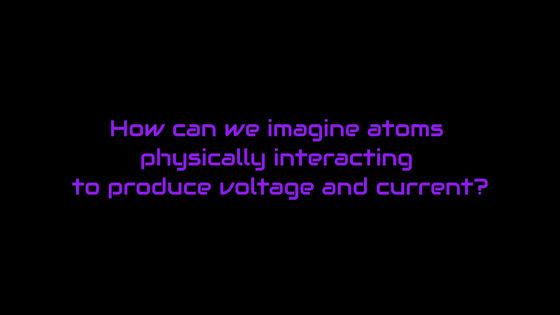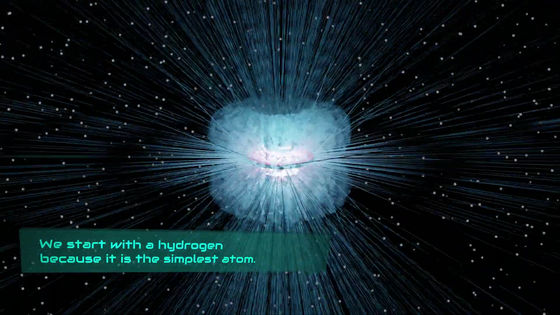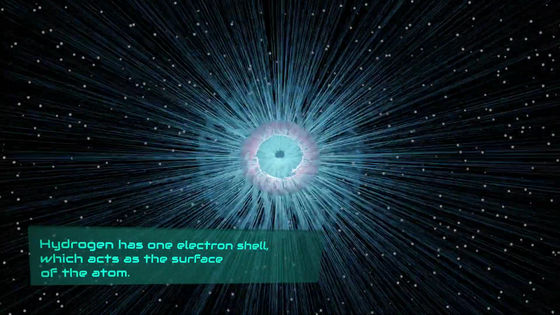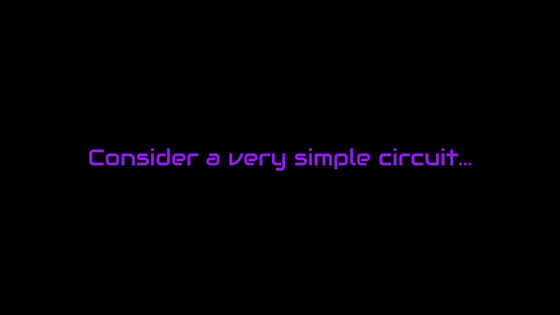# A movie that visualizes and explains 'electricity' that is usually invisibleDevices that use electricity, such as smartphones, PCs, and game consoles, are everywhere. Originally electricity is invisible to the human eye, but a movie that visualizes and explains this is published by the science blog

DEMYSTIFYING SCIENCE .

Can you imagine how the atoms physically interact to generate voltage and current? This is difficult to understand without visualizing 'invisible atomic movements.'As a first step towards that, let's first consider hydrogen, the simplest atom.Hydrogen has one

electron shell that surrounds the nucleus. The shape of this electron shell is roughly based on the radial distribution function of the electrons on the electron shell. Also, since the electron shell changes its shape depending on the excited state , this movement can be compared to human 'respiration movement'. This phenomenon is called ' quantum jump '.

99.999% of the electrons are within 430 picometers from the nucleus. However, the remaining 0.001% of electrons can be explained as a small radial filament, and there is no limit to how long it stretches.Therefore, if the radial distribution of electrons and quantum jump are ignored, it is possible to visualize electricity using a very simplified model.

So, based on the above conditions, I will consider the visualization of electricity using a 'very simple circuit diagram'.This is the 'very simple circuit diagram'.

This open circuit assumes that the hydrogen atoms are formed in a row.

In that case, the electron shells of each hydrogen atom will be entangled, and they will be lined up so that they are folded together with the adjacent hydrogen atom.

What's happening on a charged circuit?

The electric current flows from positive to negative on the circuit, but the atom on the negative side rotates counterclockwise. This rotation indicates 'charging'. In other words, whether or not it is charged can be determined from the direction in which the atom rotates.

On the other hand, on the positive side of the circuit, the atoms rotate clockwise. In this case, the positive side atoms rotate more slowly than the negative side atoms.

It can be said that the difference in the momentum and direction of rotation indicates the voltage difference between them. In other words, voltage can be expressed by the difference in momentum between electrons.

And when the circuit closes, the one with more exercise and the one with less exercise are activated.

When atoms are directly connected, momentum propagates through the electron shell at almost the speed of light. Therefore, the current propagates much faster than the electron's average velocity, the

drift velocity .

However, it is important to note that in this simplified schematic example, we are visualizing electricity with the assumption that we are using ionized hydrogen atoms. It is important to remember that the conductive metals and wires used in actual circuits have more complex electron shells.

In addition, since the electrons of metal atoms have a more complicated orbital shape than that of hydrogen atoms, metal atoms can make multipole contact with other atoms, and the visualization process becomes more complicated. ..

in Science,   Video, Posted by logu_ii